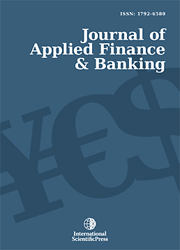# Journal of Applied Finance & Banking

#### A Recommended Financial Model for the Selection of Safest portfolio by using Simulation and Optimization Techniques

•[ Download ]
• Times downloaded: 2508
• Abstract

Investment of portfolio known that there is an important level of uncertainty about the future worth of a portfolio. The concept of value at risk (VAR) has been used to help describe a portfolio’s uncertainty. The current trend of investment in India is to invest in stock market which categorized as a high-risk level of investment. There are various methods to calculate the variance. Monte Carlo simulation method is one of the methods to calculate the VAR of the portfolio. Monte-Carlo simulation method is considered to be the optimization technique in which objective is to minimize/maximize the risk/profit before making any type of investment with portfolio. The Monte Carlo simulation method calculation for VAR of a portfolio can briefly be summarized in two steps. In the first step, a stochastic process is specified for financial variables. In the second step, financial variable of interest are simulated to get fictitious price path. The aim of the research is to develop the financial model for the safest portfolio selection based on VAR and Markowitz classical models. In the financial model, at first we measures the value at risk of Indian equity markets over short horizon of time (less than one year) by creating multiple scenarios by using Monte Carlo simulation. With the help of financial model, we ranks measured values at risk by using statistical tools. Finally, financial model will suggest an optimal portfolio over the same horizon of time using a developed optimization model. A real case study was selected and introduced to find the safest allocation of a portfolio; eight of the most active share volume was selected to perform a analysis. The results obtained by financial model indicates that the reliability description of the portfolio’s uncertainty and then gave highly reliable recommendation on portfolio optimization.ISSN: 1792-6599 (Online)
1792-6580 (Print)